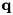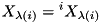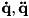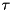Rigid Body Dynamics Library
Model Struct Reference

Contains all information about the rigid body model. More...

## Public Member Functions

Model ()

unsigned int AddBody (const unsigned int parent_id, const Math::SpatialTransform &joint_frame, const Joint &joint, const Body &body, std::string body_name="")
Connects a given body to the model. More...

unsigned int AddBodySphericalJoint (const unsigned int parent_id, const Math::SpatialTransform &joint_frame, const Joint &joint, const Body &body, std::string body_name="")

unsigned int AppendBody (const Math::SpatialTransform &joint_frame, const Joint &joint, const Body &body, std::string body_name="")
Adds a Body to the model such that the previously added Body is the Parent. More...

unsigned int AddBodyCustomJoint (const unsigned int parent_id, const Math::SpatialTransform &joint_frame, CustomJoint *custom_joint, const Body &body, std::string body_name="")

unsigned int GetBodyId (const char *body_name) const
Returns the id of a body that was passed to AddBody() More...

std::string GetBodyName (unsigned int body_id) const
Returns the name of a body for a given body id. More...

bool IsFixedBodyId (unsigned int body_id)
Checks whether the body is rigidly attached to another body. More...

bool IsBodyId (unsigned int id)

unsigned int GetParentBodyId (unsigned int id)

Math::SpatialTransform GetJointFrame (unsigned int id)

void SetJointFrame (unsigned int id, const Math::SpatialTransform &transform)

Math::Quaternion GetQuaternion (unsigned int i, const Math::VectorNd &Q) const

void SetQuaternion (unsigned int i, const Math::Quaternion &quat, Math::VectorNd &Q) const

## Data Fields

std::vector< unsigned int > lambda
The id of the parents body. More...

std::vector< unsigned int > lambda_q
The index of the parent degree of freedom that is directly influencing the current one. More...

std::vector< std::vector< unsigned int > > mu
Contains the ids of all the children of a given body. More...

unsigned int dof_count
number of degrees of freedoms of the model More...

unsigned int q_size
The size of the-vector. For models without spherical joints the value is the same as Model::dof_count, otherwise additional values for the w-component of the Quaternion is stored at the end of. More...

unsigned int qdot_size
The size of the. More...

Id of the previously added body, required for Model::AppendBody() More...

Math::Vector3d gravity
the cartesian vector of the gravity More...

std::vector< Math::SpatialVectorv
The spatial velocity of the bodies. More...

std::vector< Math::SpatialVectora
The spatial acceleration of the bodies. More...

std::vector< JointmJoints
All joints. More...

std::vector< Math::SpatialVectorS
The joint axis for joint i. More...

std::vector< Math::SpatialTransformX_J

std::vector< Math::SpatialVectorv_J

std::vector< Math::SpatialVectorc_J

std::vector< unsigned int > mJointUpdateOrder

std::vector< Math::SpatialTransformX_T
Transformations from the parent body to the frame of the joint. More...

std::vector< unsigned int > mFixedJointCount
The number of fixed joints that have been declared before each joint. More...

std::vector< Math::Matrix63multdof3_S
Motion subspace for joints with 3 degrees of freedom. More...

std::vector< Math::Matrix63multdof3_U

std::vector< Math::Matrix3dmultdof3_Dinv

std::vector< Math::Vector3dmultdof3_u

std::vector< unsigned int > multdof3_w_index

std::vector< CustomJoint * > mCustomJoints

std::vector< Math::SpatialVectorc
The velocity dependent spatial acceleration. More...

std::vector< Math::SpatialMatrixIA
The spatial inertia of the bodies. More...

std::vector< Math::SpatialVectorpA
The spatial bias force. More...

std::vector< Math::SpatialVectorU
Temporary variable U_i (RBDA p. 130) More...

Math::VectorNd d
Temporary variable D_i (RBDA p. 130) More...

Math::VectorNd u
Temporary variable u (RBDA p. 130) More...

std::vector< Math::SpatialVectorf
Internal forces on the body (used only InverseDynamics()) More...

std::vector< Math::SpatialRigidBodyInertiaI
The spatial inertia of body i (used only in CompositeRigidBodyAlgorithm()) More...

std::vector< Math::SpatialRigidBodyInertiaIc

std::vector< Math::SpatialVectorhc

std::vector< Math::SpatialVectorhdotc

std::vector< Math::SpatialTransformX_lambda
Transformation from the parent body to the current bodystd::vector< Math::SpatialTransformX_base
Transformation from the base to bodies reference frame. More...

std::vector< FixedBodymFixedBodies
All bodies that are attached to a body via a fixed joint. More...

unsigned int fixed_body_discriminator
Value that is used to discriminate between fixed and movable bodies. More...

std::vector< BodymBodies
All bodies 0 ... N_B, including the base. More...

std::map< std::string, unsigned int > mBodyNameMap
Human readable names for the bodies. More...

## Detailed Description

Contains all information about the rigid body model.

This class contains all information required to perform the forward dynamics calculation. The variables in this class are also used for storage of temporary values. It is designed for use of the Articulated Rigid Body Algorithm (which is implemented in ForwardDynamics()) and follows the numbering as described in Featherstones book.

Please note that body 0 is the root body and the moving bodies start at index 1. This numbering scheme is very beneficial in terms of readability of the code as the resulting code is very similar to the pseudo-code in the RBDA book. The generalized variables q, qdot, qddot and tau however start at 0 such that the first entry (e.g. q) always specifies the value for the first moving body.

Note
To query the number of degrees of freedom use Model::dof_count.

Definition at line 121 of file Model.h.

## ◆ Model()

 Model ( )

Definition at line 23 of file Model.cc.

## Member Function Documentation

 unsigned int AddBody ( const unsigned int parent_id, const Math::SpatialTransform & joint_frame, const Joint & joint, const Body & body, std::string body_name = "" )

Connects a given body to the model.

When adding a body there are basically informations required:

• what kind of body will be added?
• where is the new body to be added?
• by what kind of joint should the body be added?

The first information "what kind of body will be added" is contained in the Body class that is given as a parameter.

The question "where is the new body to be added?" is split up in two parts: first the parent (or successor) body to which it is added and second the transformation to the origin of the joint that connects the two bodies. With these two informations one specifies the relative positions of the bodies when the joint is in neutral position.gk

The last question "by what kind of joint should the body be added?" is again simply contained in the Joint class.

Parameters
 parent_id id of the parent body joint_frame the transformation from the parent frame to the origin of the joint frame (represents X_T in RBDA) joint specification for the joint that describes the connection body specification of the body itself body_name human readable name for the body (can be used to retrieve its id with GetBodyId())
Returns

Definition at line 253 of file Model.cc.

 unsigned int AddBodyCustomJoint ( const unsigned int parent_id, const Math::SpatialTransform & joint_frame, CustomJoint * custom_joint, const Body & body, std::string body_name = "" )

Definition at line 475 of file Model.cc.

 unsigned int AddBodySphericalJoint ( const unsigned int parent_id, const Math::SpatialTransform & joint_frame, const Joint & joint, const Body & body, std::string body_name = "" )

## ◆ AppendBody()

 unsigned int AppendBody ( const Math::SpatialTransform & joint_frame, const Joint & joint, const Body & body, std::string body_name = "" )

Adds a Body to the model such that the previously added Body is the Parent.

This function is basically the same as Model::AddBody() however the most recently added body (or body 0) is taken as parent.

Definition at line 463 of file Model.cc.

## ◆ GetBodyId()

 unsigned int GetBodyId ( const char * body_name ) const
inline

Returns the id of a body that was passed to AddBody()

Bodies can be given a human readable name. This function allows to resolve its name to the numeric id.

Note
Instead of querying this function repeatedly, it might be advisable to query it once and reuse the returned id.
Returns
the id of the body or std::numeric_limits<unsigned int>::max() if the id was not found.

Definition at line 346 of file Model.h.

## ◆ GetBodyName()

 std::string GetBodyName ( unsigned int body_id ) const
inline

Returns the name of a body for a given body id.

Definition at line 355 of file Model.h.

## ◆ GetJointFrame()

 Math::SpatialTransform GetJointFrame ( unsigned int id )
inline

Returns the joint frame transformtion, i.e. the second argument to Model::AddBody().

Definition at line 415 of file Model.h.

## ◆ GetParentBodyId()

 unsigned int GetParentBodyId ( unsigned int id )
inline

Determines id the actual parent body.

When adding bodies using joints with multiple degrees of freedom, additional virtual bodies are added for each degree of freedom. This function returns the id of the actual non-virtual parent body.

Definition at line 398 of file Model.h.

## ◆ GetQuaternion()

 Math::Quaternion GetQuaternion ( unsigned int i, const Math::VectorNd & Q ) const
inline

Gets the quaternion for body i (only valid if body i is connected by a JointTypeSpherical joint)

See Joint Singularities for details.

Definition at line 461 of file Model.h.

References RigidBodyDynamics::JointTypeSpherical.

## ◆ IsBodyId()

 bool IsBodyId ( unsigned int id )
inline

Definition at line 380 of file Model.h.

## ◆ IsFixedBodyId()

 bool IsFixedBodyId ( unsigned int body_id )
inline

Checks whether the body is rigidly attached to another body.

Definition at line 371 of file Model.h.

## ◆ SetJointFrame()

 void SetJointFrame ( unsigned int id, const Math::SpatialTransform & transform )
inline

Sets the joint frame transformtion, i.e. the second argument to Model::AddBody().

Definition at line 435 of file Model.h.

## ◆ SetQuaternion()

 void SetQuaternion ( unsigned int i, const Math::Quaternion & quat, Math::VectorNd & Q ) const
inline

Sets the quaternion for body i (only valid if body i is connected by a JointTypeSpherical joint)

See Joint Singularities for details.

Definition at line 476 of file Model.h.

References RigidBodyDynamics::JointTypeSpherical.

## ◆ a

 std::vector a

The spatial acceleration of the bodies.

Definition at line 168 of file Model.h.

## ◆ c

 std::vector c

The velocity dependent spatial acceleration.

Definition at line 208 of file Model.h.

## ◆ c_J

 std::vector c_J

Definition at line 182 of file Model.h.

## ◆ d

Temporary variable D_i (RBDA p. 130)

Definition at line 216 of file Model.h.

## ◆ dof_count

 unsigned int dof_count

number of degrees of freedoms of the model

This value contains the number of entries in the generalized state (q) velocity (qdot), acceleration (qddot), and force (tau) vector.

Definition at line 139 of file Model.h.

## ◆ f

 std::vector f

Internal forces on the body (used only InverseDynamics())

Definition at line 220 of file Model.h.

## ◆ fixed_body_discriminator

 unsigned int fixed_body_discriminator

Value that is used to discriminate between fixed and movable bodies.

Bodies with id 1 .. (fixed_body_discriminator - 1) are moving bodies while bodies with id fixed_body_discriminator .. max (unsigned int) are fixed to a moving body. The value of max(unsigned int) is determined via std::numeric_limits<unsigned int>::max() and the default value of fixed_body_discriminator is max (unsigned int) / 2.

On normal systems max (unsigned int) is 4294967294 which means there could be a total of 2147483646 movable and / or fixed bodies.

Definition at line 254 of file Model.h.

## ◆ gravity

 Math::Vector3d gravity

the cartesian vector of the gravity

Definition at line 162 of file Model.h.

## ◆ hc

 std::vector hc

Definition at line 225 of file Model.h.

## ◆ hdotc

 std::vector hdotc

Definition at line 226 of file Model.h.

## ◆ I

 std::vector I

The spatial inertia of body i (used only in CompositeRigidBodyAlgorithm())

Definition at line 223 of file Model.h.

## ◆ IA

 std::vector IA

The spatial inertia of the bodies.

Definition at line 210 of file Model.h.

## ◆ Ic

 std::vector Ic

Definition at line 224 of file Model.h.

## ◆ lambda

 std::vector lambda

The id of the parents body.

Definition at line 127 of file Model.h.

## ◆ lambda_q

 std::vector lambda_q

The index of the parent degree of freedom that is directly influencing the current one.

Definition at line 130 of file Model.h.

## ◆ mBodies

 std::vector mBodies

All bodies 0 ... N_B, including the base.

mBodies - base body
mBodies - 1st moveable body
...
mBodies[N_B] - N_Bth moveable body

Definition at line 263 of file Model.h.

## ◆ mBodyNameMap

 std::map mBodyNameMap

Human readable names for the bodies.

Definition at line 266 of file Model.h.

## ◆ mCustomJoints

 std::vector mCustomJoints

Definition at line 202 of file Model.h.

## ◆ mFixedBodies

 std::vector mFixedBodies

All bodies that are attached to a body via a fixed joint.

Definition at line 241 of file Model.h.

## ◆ mFixedJointCount

 std::vector mFixedJointCount

The number of fixed joints that have been declared before each joint.

Definition at line 191 of file Model.h.

## ◆ mJoints

 std::vector mJoints

All joints.

Definition at line 175 of file Model.h.

## ◆ mJointUpdateOrder

 std::vector mJointUpdateOrder

Definition at line 184 of file Model.h.

## ◆ mu

 std::vector > mu

Contains the ids of all the children of a given body.

Definition at line 132 of file Model.h.

## ◆ multdof3_Dinv

 std::vector multdof3_Dinv

Definition at line 198 of file Model.h.

## ◆ multdof3_S

 std::vector multdof3_S

Motion subspace for joints with 3 degrees of freedom.

Definition at line 196 of file Model.h.

## ◆ multdof3_U

 std::vector multdof3_U

Definition at line 197 of file Model.h.

## ◆ multdof3_u

 std::vector multdof3_u

Definition at line 199 of file Model.h.

## ◆ multdof3_w_index

 std::vector multdof3_w_index

Definition at line 200 of file Model.h.

## ◆ pA

 std::vector pA

The spatial bias force.

Definition at line 212 of file Model.h.

Id of the previously added body, required for Model::AppendBody()

Definition at line 159 of file Model.h.

## ◆ q_size

 unsigned int q_size

The size of the-vector. For models without spherical joints the value is the same as Model::dof_count, otherwise additional values for the w-component of the Quaternion is stored at the end of.

Joint Modeling for more details.

Definition at line 148 of file Model.h.

## ◆ qdot_size

 unsigned int qdot_size

The size of the.

(, and-vector.

Joint Modeling for more details.

Definition at line 156 of file Model.h.

## ◆ S

 std::vector S

The joint axis for joint i.

Definition at line 177 of file Model.h.

## ◆ U

 std::vector U

Temporary variable U_i (RBDA p. 130)

Definition at line 214 of file Model.h.

## ◆ u

Temporary variable u (RBDA p. 130)

Definition at line 218 of file Model.h.

## ◆ v

 std::vector v

The spatial velocity of the bodies.

Definition at line 166 of file Model.h.

## ◆ v_J

 std::vector v_J

Definition at line 181 of file Model.h.

## ◆ X_base

 std::vector X_base

Transformation from the base to bodies reference frame.

Definition at line 238 of file Model.h.

## ◆ X_J

 std::vector X_J

Definition at line 180 of file Model.h.

## ◆ X_lambda

 std::vector X_lambda

Transformation from the parent body to the current body.

Definition at line 236 of file Model.h.

## ◆ X_T

 std::vector X_T

Transformations from the parent body to the frame of the joint.

Definition at line 188 of file Model.h.

The documentation for this struct was generated from the following files: